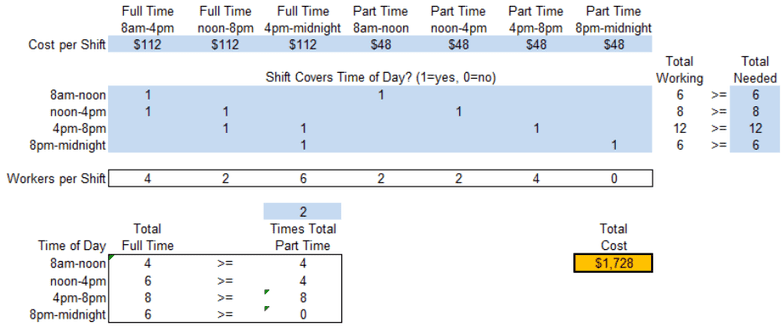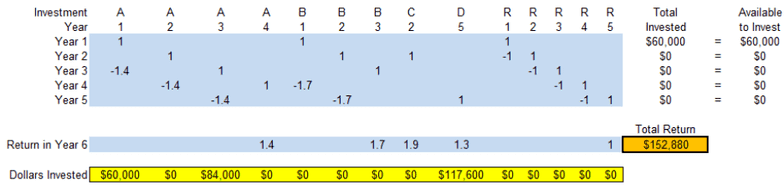Textbook Notes (280,000)
CA (170,000)
UOttawa (6,000)
Chapter 4

# ADM 2302 Chapter Notes - Chapter 4: Lincoln Near-Earth Asteroid Research, Chief Operating Officer, List Of Subaru Engines

Department
Course Code
Professor
Rim Jaber
Chapter
4

This preview shows pages 1-2. to view the full 7 pages of the document.1
Week 4 Extra Problems Solution
CHAPTER 3
LINEAR PROGRAMMING: FORMULATION AND
APPLICATIONS
Problems
3.16 a) This is a cost-benefit-tradeoff problem because it asks you to meet minimum required
benefit levels (number of consultants working each time period) at minimum cost.
b)
c) Let f1 = number of full-time consultants working the morning shift (8 a.m.-4 p.m.),
f2 = number of full-time consultants working the afternoon shift (12 p.m.-8 p.m.),
f3 = number of full-time consultants working the evening shift (4 p.m.-midnight),
p1 = number of part-time consultants working the first shift (8 a.m.-12 p.m.),
p2 = number of part-time consultants working the second shift (12 p.m.-4 p.m.),
p3 = number of part-time consultants working the third shift (4 p.m.-8 p.m.),
p4 = number of part-time consultants working the fourth shift (8 p.m.-midnight).

Only pages 1-2 are available for preview. Some parts have been intentionally blurred.2
Minimize C = (\$14 / hour)(8 hours)(f1 + f2 + f3) + (\$12/ hour)(4 hours)(p1 + p2 + p3 + p4)
subject to
f1 + p1 6
f1 + f2 + p2 ≥ 8
f2 + f3 + p3 12
f3 + p4 ≥ 6
f1 ≥ 2p1
f1 + f2 ≥ 2p2
f2 + f3 ≥ 2p3
f3 2p4
and f1 0, f2 0, f3 0, p1 ≥ 0, p2 0, p3 0, p4 0.
3.19 a) A1 + B1 + R1 = \$60,000
A2 + B2 + C2 + R2 = R1
A3 + B3 + R3 = R2 + 1.40A1
A4 + R4 = R3 + 1.40A2 + 1.70B1
D5 + R5 = R4 + 1.40A3 + 1.70B2
b) Let At = amount invested in investment A at the beginning of year t.
Bt = amount invested in investment B at the beginning of year t.
Ct = amount invested in investment C at the beginning of year t.
Dt = amount invested in investment D at the beginning of year t.
Rt = amount not invested at the beginninf of year t.
Maximize Return = 1.40A4 + 1.70B3 + 1.90C2 + 1.30D5 + R5
subject to A1 + B1 + R1 = \$60,000
A2 + B2 + C2 R1 + R2 = 0
1.40A1 + A3 + B3 R2 + R3 = 0
1.40A2 + A4 1.70B1 R3 + R4 = 0
1.40A3 1.70B2 + D5 R4 + R5 = 0
and At 0, Bt ≥ 0, Ct 0, Dt 0, Rt ≥ 0.
c)